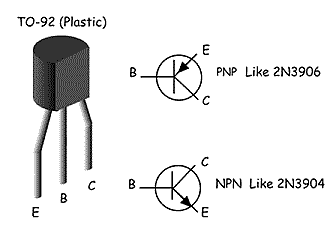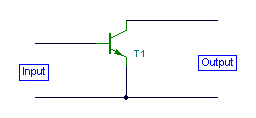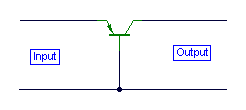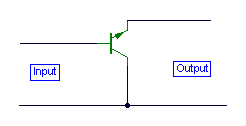Technicial and Operating Info:

Eelectronic component symbols.

Electronic formulas
.

Capacitor stuff:

Capacitor chart.

Capacitance: A measure of the energy storage ability of a capacitor, given as C = K A/D, where A is the area of the electrodes, D is their separation, and K is a function of the dielectric between the electrodes. The formula yields a result in farads (F ), but a farad is so large that the most commonly used values are expressed in microfarads ( µf = 10-6F ) or picofarads ( pf = 10-12F ).

Working voltage (Wvdc, Wvac): The maximum continuous voltage that should be applied to a capacitor. Rated voltages for DC and AC operation are usually not the same.

Temperature Coefficient (TC): The change in capacitance with temperature expressed linearly as parts per million per degree centigrade (PPM/°C), or as a percent change over a specified temperature range. Most film capacitors are not linear and TC is expressed in percent.

Resistor chart.

Torroid (iron powder & ferrite) info.

Transister stuff:
 Transistor PinoutsHere's a very common plastic package; the TO-92. Beware, not all parts in TO-92 share this pinout. Here are some that do: 2N3903, 2N3904, 2N3905, 2N3906 2N4400, 2N4401, 2N4402, 2N4403

There are plenty of texts around on basic electronics, so this is a very brief look at the three basic ways in which a bipolar junction transistor (BJT) can be used. In each case, one terminal is common to both the input and output signal. All the circuits shown here are without bias circuits and power supplies for clarity.

Common Emitter ConfigurationHere the emitter terminal is common to both the input and output signal. The arrangement is the same for a PNP transistor. Used in this way the transistor has the advantages of a medium input impedance, medium output impedance, high voltage gain and high current gain.

Common Base ConfigurationHere the base is the common terminal. Used frequently for RF applications, this stage has the following properties. Low input impedance, high output impedance, unity (or less) current gain and high voltage gain.

Common Collector ConfigurationThis last configuration is also more commonly  known as the emitter follower. This is because the input signal applied at the base is "followed" quite closely at the emitter with a voltage gain close to unity. The properties are a high input impedance, a very low output impedance, a unity (or less) voltage gain and a high current gain. This circuit is also used extensively as a "buffer" converting impedances or for feeding or driving long cables or low impedance loads.

A note on Phase Shifts
In both the the common emitter and emitter follower configurations, the input and output signals are out of phase by 180 degrees. This is not a phase displacement, but a phase inversion. The common base mode however tracks the input and output with 0 degrees phase shift.

Transistor / IC / Diode layout.

DXCC Country list.